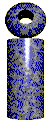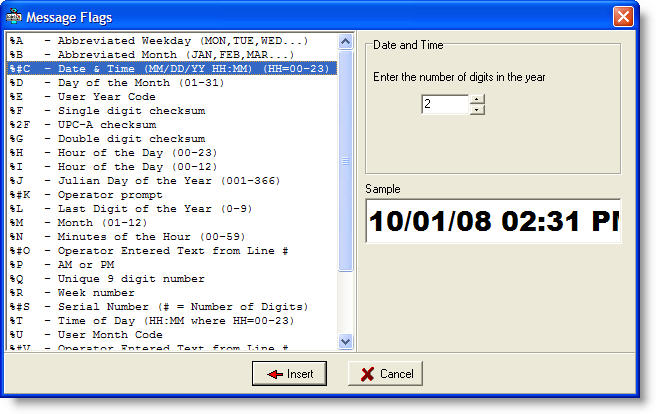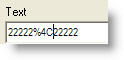# LabeLase® Producer Special Flags and Functions

## Special Flag Fields

Special flags are available that cause the printer to automatically insert text into a field. These flags can be inserted anywhere in the text string. All flags begin with a percent sign “%” and are followed by an optional number and then a character.

The following flags are available for use. Note that the computer’s locale setting may affect the output of these flags, including language, order, etc.

 %A Abbreviated Weekday (MON, TUE, WED...) %B Abbreviated Month (JAN, FEB, MAR...) %C Date & Time (date is short date format, time is long time format) %D Day of the Month (01-31) %E User Year Code %F Single Digit Checksum %2F Single Digit Checksum for UPC-A barcodes (new in V1.30) %G Double Character Checksum %H Hour of the Day (00-23) %I Hour of the Day (00-12) %J Julian Day of the Year (001-366) %#K Operator Prompt from Line # %L Last Digit of the Year (0-9) %M Month (01-12) %N Minutes of the Hour (00-59) %P AM or PM %Q A unique number which changes every print. %R Week number of the year %#S# Serial Number (1st # = Number of Digits, 2nd # = S/N Field ID) %T Time of Day (short time format HH:MM:SS AM) %U User Month Code %#V Operator Entered Text from Line # %W Weekday Number (1=Sunday to 7=Saturday) %X Date (MM/DD/YYYY) %Y Year (00-99) %Z User Shift Code %#\$ Link to Text Field ID #

Any automatic text flag may be followed by a “sub-string specification” which can be used to extract a portion of the automatically generated text. Following the flag with this sequence specifies the sub-string:

(#, #)

The first number following the open parenthesis character specifies the character position of the first character to be included. The first character in the string is character number 1.

The second number following the comma specifies the number of characters to include in the text.

For example, let’s say that the %X(1, 5) flag is included in the message and the date is 03/26/2001. The sub-string that will be included in the message is 03/26 because it will begin with the first character and include 5 characters.

Flag Help Form

If you click the Flag Help button in the Text Field Editor form, the form shown below will appear, which provides help on the various flags that can be inserted into the message.The left pane of the form shows a list of the flags that are available to you for insertion into your text field.  When you select a flag, the right pane shows a preview sample of the text that will be generated by the flag.   Some flags have optional parameters that you can specify as well.   In the above exampe, the %C flag can have an optional number before the letter C that specifies the number of digits to put in the year portion of the generated text.   In this example, you may specify 2 or 4 digits.

Once you have selected your flag and provided the optional parameter for it, you may click the Insert button.   The form will close, and the selected flag will be inserted into your text message at the cursor.Text field before using the Insert feature of the Flag Help form.  Note the position of the cursor within the text.   This is where the flag will be placed.Text field after inserting the %4C flag from the Flag Help Form

## Functions

New in V1.90 is a string function processor that allows you to perform string, mathematical and logical operations on text fields or barcode contents.

The function evaluator provided in V1.90 and later of Producer is specifically designed for text string processing.   All function arguments and results are text strings by their nature, although some functions interpret strings as numbers and some return results are numbers represented as strings.

Functions can be embedded anywhere within a text string, and upon evaluation, will be replaced by the result of the function computation.  Any number of functions can be included in a single text field, and functions may be nested to any level.

Each function begins with a special sequence that identifies the start of a function.  This sequence is the combination of a colon and an equal sign ":=".   Immediately following this start sequence is the name of the function.   All function names are followed by an argument list with zero or more arguments enclosed in parentheses.

For example, the "concat" function simply returns its argument list combined (or concatenated) as a single string as shown below (note that in all examples, double quotes enclose the strings for clarity only and are not required):

Input Text Result
`"ABC :=concat(Hello,World) DEF"`
`"ABC HelloWorld DEF"`
`"ABC :=concat(Hello, World) DEF"`
`"ABC Hello World DEF"`
`"ABC :=concat(Hello,concat( World)) DEF"`
`"ABC Hello World DEF"`
`"ABC :=concat(Hello,( World)) DEF"`
`"ABC Hello World DEF"`

Notice that in all examples, the function is surrounded by text strings "ABC" and  "DEF" that are copied directly to the output.   In the first example, the arguments to the concat function are placed directly next to each other with no intervening space.   This is because the second argument immediately follows the comma, which separates the arguments.   The second example corrects this by placing a comma before the word "World".

The third example above produces the same same result as the second, but is perhaps more obvious since the space character is part of the argument to the second, nested concat function.

The fourth example also produces the desired result by using the anonymous function - simply leave off the function name - which is the same as the concat function.

Function Arguments

Functions take zero or more arguments.   Functions will ignore arguments beyond their required number, except for those functions that take an unlimited number of arguments.

Arguments are separated from each other by commas.   Spaces within arguments are significant as shown in the examples above, unless the argument is itself a function call.   For example, in a reworking of the third example above, the added spaces before the nested concat function call are ignored and not part of the output result:

Input Text Result
`"ABC :=concat(Hello,      concat( World)) DEF"`
`"ABC Hello World DEF"`

If you don't supply the minimum number of arguments for a function, you will receive an error flag in the result text.

Argument Types

The function processor is designed to handle text strings, so all arguments are fundamentally just text.   However, some functions treat text strings as numbers or logical values.

Argument Type Example
Integer "123"
Decimal "12.34"
Logical "0" = False, "1" = True
String "ABC123"

Return Results

Each function returns a result that is a text string by nature.   However, just like function arguments, return results may be interpreted as numbers or logical values depending on the function.

The return result of the outer level function is always embedded into the surrounding text, even if the return result is a null string (empty).

Error Messages

If you have an error in your formula, the processor will insert an error message into the result string.   The error message begins and ends with an exclamation point.   If the error is specific to a function, the function name will follow the first exclamation point.   The type of error follows the function name.

Error Message Meaning
!DIV0! Division by zero
!NUMARGS! Invalid number of arguments
!?FUNC! Unknown function name
!PAREN! Mismatched parentheses

Function Reference

The following functions are supported by Producer for both text and barcode fields:

String Functions

Name Arguments Returns Example
asc(ch)
Return the ASCII value for the character ch
ch - string (only the first character is used) integer ":=asc(A)"
returns "65"
char(value)
Return the character whose ASCII value is <value>
value - integer string ":=char(65)"
returns "A"
concat(arg1,arg2,...argN)
Return a string that is the concatenation of all arguments
one or more text strings string ":=concat(ABC,123)"
returns "ABC123"
dformat(value,width,precision)
Format the decimal value as a string
value - decimal value
width - minimum number of characters for resulting text - padded as needed
precision - number of places after the decimal point
string ":=dformat(1.2345,1,2)"
returns "1.23"

":=dformat(10.0,10,3)"
returns "    10.000"

empty()
Return an empty string
none string ":=empty()"
returns ""
iformat(value,width,precision)
Format the integer value as a string
value - integer value
width - minimum number of characters for resulting text - padded as needed - optional
precision - minimum number of digits - optional
string ":=iformat(10,3)"
returns "10"

":=iformat(10,3,3)"
returns "010"

isempty(arg1,...argN)
Return True "1" if all arguments are empty strings, else returns False "0"
one or more text strings logical "0" or "1" ":=isempty()"
returns "1"

":=isempty(A)"
returns "0"

":=isempty(empty())"
returns "1"

left(text,count)
Return the leftmost <count> characters from <text>
text - a text string
count - the number of characters
string ":=left(InfoSight, 4)"
returns "Info"
lower(text)
Return text converted to all lower case
text - a text string string ":=lower(InfoSight)"
returns "infosight"
proper(text)
Return text converted to proper case
text - a text string string ":=proper(hello world)"
returns "Hello World"
replace(text,old,new)
Replace all occurrences of <old> with <new> in <text>
text - a text string
old - the text to be replaced
new - the replacement text
string ":=replace(ABCD,BC,X)"
returns "AXD"
rept(text,count)
Return a string that is <string> repeated <count> times
text - a text string
count - number of repetitions
string ":=rept(AB,4)"
returns "ABABABAB"
right(text,count)
Return the rightmost <count> characters from <text>
text - a text string
count - the number of characters
string ":=right(InfoSight, 5)"
returns "Sight"
streq(text1,text2,...textN)
Return True "1" if all text string arguments are equal
one or more text strings logical ":=streq(AB,CD)"
returns "0"

":=streq(AB,AB,AB)"
returns "1"

strgt(text1,text2)
Returns True "1" if <text1> is greater than <text2>
text1 - a text string
text2 - a text string
logical ":=strgt(C,B)"
returns "1"

":=strgt(B,B)"
returns "0"

strlen(text)
Returns the length (number of characters) of <text>
text - a text string integer ":=strlen(ABC)"
returns "3"
strlt(text1, text2)
Returns True "1" if <text1> is less than <text2>
text1 - a text string
text2 - a text string
logical ":=strlt(A,B)"
returns "1"

":=strlt(B,B)"
returns "0"

strpos(text1, text2)
Returns the position within <text1> of the string <text2> where 1 is the first character.
text1 - the text string to search
text2 - the text string to find
integer ":=strpos(InfoSight,Si)"
returns "5"
substr(text,start,length)
Returns a sub-string of <text> starting at position <start> where 1 is the first character, containing at most <length> characters
text - a text string
start - integer starting character position
length - number of characters
string ":=substr(InfoSight,3,2)"
returns "fo"
trim(text)
Remove leading and trailing whitespace from <text>
text - a text string string ":=trim(   Hello   )"
returns "Hello"

Mathematical Functions

Name Arguments Returns Example
abs(number)
Returns the absolute value of <number>
number - integer or decimal decimal ":=abs(5)"
returns "5"

":=abs(-5)"
returns "5"

avg(num1,num2,...numN)
Returns the average of all argument
one or more numbers, integer or decimal decimal ":=avg(25.0,33.5,17,44.1)"
returns "29.9"
ceil(number)
Returns the smallest integer not less than <number>
number - decimal number integer ":=ceil(210.67)"
returns "211"

":=ceil(-4.5)"
returns "-4"

div(num1,num2)
Returns the quotient from the division of <num1> by <num2>
num1 - integer or decimal number
num2 - integer or decimal number
decimal ":=div(10,5)"
returns "2"
eq(num1,num2,prec)
Returns True "1" if <num1> is equal to <num2> within precision <prec>
num1 - decimal number
num2 - decimal number
prec - decimal precision value - optional default = 0.000001
logical ":=eq(5,5.00001)"
returns "0"

":=eq(5,5.0000001)"
returns "1"

floor(number)
Returns the largest integer not greater than <number>
number - a decimal number integer ":=floor(169.65)"
returns "169"

":=floor(-14.32)"
returns "-15"

gt(num1,num2)
Returns True "1" if <num1> is numerically greater than <num2>
num1 - integer or decimal
num2 - integer or decimal
logical ":=gt(1.2,1.09)"
returns "1"

":=gt(-5,-3)"
returns "0"

lt(num1,num2)
Returns True "1" if <num1> is numerically less than <num2>
num1 - integer or decimal
num2 - integer or decimal
logical ":=gt(1.2,1.09)"
returns "0"

":=gt(-5,-3)"
returns "1"

max(num1,num2,...numN)
Return the maximum number in the list of arguments
one or more numbers decimal ":=max(10,14,19,6,4,2)"
returns "19"

":=max(10,14,14.01,6,4,2)"
returns "14.01"

min(num1,num2,...numN)
Return the minimum number in the list of arguments
one or more numbers decimal ":=min(10,14.9,14.9001,4,2)"
returns "2"

":=min(10,14,-19,6,4,2)"
returns "-19"

mod(num1,num2)
Returns the integer remainder after dividing <num1> by <num2>
num1 - integer number
num2 - integer number
integer ":=mod(10,7)"
returns "3"
mult(num1,num2,...numN)
Returns the product of all arguments
one or more numbers decimal ":=mult(10,5)"
returns "50"
pow(number,exp)
Returns <number> raised to the <exp> power.
number - an integer or decimal
exp - the exponent
decimal ":=pow(4,2)"
returns "16"

":=pow(2.5,2)"
returns "6.25"

sqrt(number)
Returns the square root of <number>
number - an integer or decimal decimal ":=sqrt(25)"
returns "5"
subtract(num1, num2)
Returns the difference <num1> minus <num2>
num1 - an integer or decimal
num2 - an integer or decimal
decimal ":=subtract(10,3)"
returns "7"
sum<num1,num2,...numN)
Returns the numerical summation of all arguments
one or more numbers decimal ":=sum(1,2,3,4,5)"
returns "15"

Logical Functions

Name Arguments Returns Example
and(arg1,arg2,...argN)
Returns True "1" only if all arguments are non-zero
one or more numeric or logical logical ":=and(1,1,1,0)"
returns "0"

":=and(1,1,1,1)"
returns "1"

false()
Returns "0"
none logical ":=false()"
returns "0"
if(test,true,false)
Returns the argument <true> if the value of <test> is non-zero, else returns the argument <false>
test - numeric or logical test
true - argument to return if <test> is non-zero
false - argument to return if <test> is zero
any ":=if(0,True,False)"
returns "False"

":=if(gt(5.0,4.9),True,False)"
returns "True"

not(arg)
Returns the logical negation of <arg>.  If <arg> is zero, returns "1", else "0"
arg - numeric or logical logical ":=not(true())"
returns "0"
or(arg1,arg2,...argN)
Returns True "1" if any arguments are non-zero
one or more numeric or logical logical ":=or(0,0,0,1)"
returns "1"

":=or(0,0,0,0)"
returns "0"

true()
Returns "1"
none logical ":=true()"
returns "1"

Miscellaneous Functions

Name Arguments Results Example
gs1cksum(text)
Return <text> as a GS1 barcode symbology AI (01) string including the checksum
text - 13 digits representing an AI(01) identifier string ":=gs1cksum(1801437557290)"
returns "18014375572900"
ft_to_m(number)
Return <number> converted from feet to meters
number - an integer or decimal decimal ":=ft_to_m(5.0)"
returns "1.524"
m_to_ft(number)
Returns <number> converted from meters to feet
number - an integer or decimal decimal ":=m_to_ft(12.0)"
returns "39.3700787401575"
lb_to_kg(number)
Returns <number> converted from pounds to kilograms
number - an integer or decimal decimal ":=lb_to_kg(3.0)"
returns "1.36077718520971"
kg_to_lb(number)
Returns <number> converted from kilograms to pounds
number - an integer or decimal decimal ":=kg_to_lb(5.0)"
returns "11.0231125"